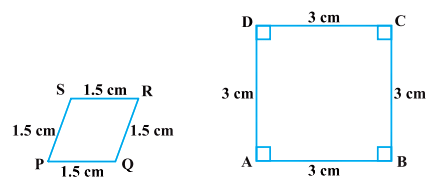NCERT Solutions: Triangles (Exercise 6.1)

# NCERT Solutions: Triangles (Exercise 6.1) - Notes | Study Mathematics (Maths) Class 10 - Class 10

 Table of contentsExercise 6.11 Crore+ students have signed up on EduRev. Have you?

Exercise 6.1

Q1. Fill in the blanks using the correct word given in brackets.
(i) All circles are _______(congruent, similar).
(ii) All squares are _______ (similar, congruent).
(iii) All _______ triangles are similar. (isosceles, equilateral).
(iv)Two polygons of the same number of sides are similar, if
(a) their corresponding angles are _______ and
(b) their corresponding sides are _______ (equal, proportional).
Ans: (i) All circles are similar.
(ii) All squares are similar.
(iii) All equilateral triangles are similar.
(iv) Two polygons of the same number of sides are similar if:
(a) Their corresponding angles are equal and
(b) Their corresponding sides are proportional.

Q2. Give two different examples of pair of:
(i) similar figures
(ii) non-similar figures.
Ans:
(i).
(a) Any two circles are similar figures.
(b) Any two squares are similar figures.
(ii).
(a) A circle and a triangle are non-similar figures.
(b) An isosceles triangle and a scalene triangle are non-similar figures.

Q3. State whether the following quadrilaterals are similar or not.Ans: No, the sides of quadrilateral PQRS and ABCD are proportional but their corresponding angles are not equal.
∴ These are not similar.

The document NCERT Solutions: Triangles (Exercise 6.1) - Notes | Study Mathematics (Maths) Class 10 - Class 10 is a part of the Class 10 Course Mathematics (Maths) Class 10.
All you need of Class 10 at this link: Class 10

## Mathematics (Maths) Class 10

53 videos|403 docs|138 tests
 Use Code STAYHOME200 and get INR 200 additional OFF

## Mathematics (Maths) Class 10

53 videos|403 docs|138 tests

Track your progress, build streaks, highlight & save important lessons and more!

,

,

,

,

,

,

,

,

,

,

,

,

,

,

,

,

,

,

,

,

,

;